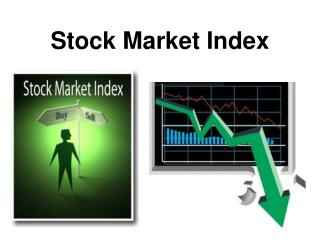# Stock Market Index - PowerPoint PPT PresentationDownload PresentationStock Market Index

Stock Market IndexDownload Presentation## Stock Market Index

- - - - - - - - - - - - - - - - - - - - - - - - - - - E N D - - - - - - - - - - - - - - - - - - - - - - - - - - -
##### Presentation Transcript

1. Stock Market Index

2. Definition • A stock market index is a hypothetical basket of securities designed to track market changes. • The most widely followed US stock market indexes are: • DJIA • S&P 500 • NASDAQ Composite Important international stock market indexes are the Nikkei 225 (Japan)

3. Index differ from one another with respect to: • The securities included in the index and • The method used in calculating the value of index

4. Types • Price-Weighted Index • Value –Weighted Index • Equal- Weighted • Geometric Mean

5. Price-Weighted Index • Definition: • Suppose there are two stocks A & B • Average = A+B / d (Where d is divisor) • Formula: I1 = I o * AP1 /APo

6. Adjusting the Divisor with stock Split • At T1 B splits (two of one) • Number of share has increased • To Price: 20 • T1 Price: 11 -------- (*2)= 22

7. Value –Weighted Index • Definition: • Suppose there are two stocks A & B • Formula: I1 = I o * MV1 /MV0

8. Equal–Weighted Index Definition: This index is computed by multiplying the level of the index on the previous day by the arithmetic mean of the daily price relatives of the relevant stocks in the index. Daily Price relativesToday’s price dived by yesterday’s price • Suppose there are two stocks A & B

9. Calculation • Suppose there are two stocks A & B • A: 13 / 10 = 1.3 • B: 22 / 20 = 1.1 Now calculate the arithmetic mean • 1.3 + 1.1 / 2 = 1.20

10. Consider the value of index on day To =100 Then , I1 =Arithmetic Mean*I0 I1 = 1.20 * 100 = 120 *The value line Composite (Arithmetic) index, based on more than 1500 stocks is prepared in this manner

11. Geometric Mean Index Definition: This index is computed by multiplying the level of the index on the previous day by the geometric mean of the daily price relatives of the relevant stocks in the index. • Suppose there are two stocks A & B

12. Geometric Mean Index • Definition: • Suppose there are two stocks A & B • A: 13 / 10 = 1.3 • B: 22 / 20 = 1.1 • Now calculate the Geometric mean • (1.3 * 1.1) = 1.1958 1/2

13. Consider the value of index on day To =100 • Then , • I1 = I0 * Geometric mean • I1 = 100 * 1.1958 • = 119.58

14. Comparing the Indices • P-W I = 16.67 % • V-W I = 15.45 % • E-W I = 20 % • GM = 19.58 %

15. Stock Split • A corporate action in which a company's existing shares are divided into multiple shares. • The number of shares outstanding increases by a specific multiple, the total dollar value of the shares remains the same compared to pre-split amounts. • Because no real value has been added as a result of the split.In the U.K., a stock split is referred to as: • "scrip issue", "bonus issue", "capitalization issue" or "free issue".

16. For example, in a 2-for-1 split, each stockholder receives an additional share for each share he or she holds.Why stock splits are performed? • A company's share price has grown so high that to many investors, the shares are too expensive to buy in round lots. For example, if a XYZ Corp.'s shares were worth \$1,000 each • Investors would need to purchase \$100,000 in order to own 100 shares. • If each share was worth \$10, investors would only need to pay \$1,000 to own 100 shares.